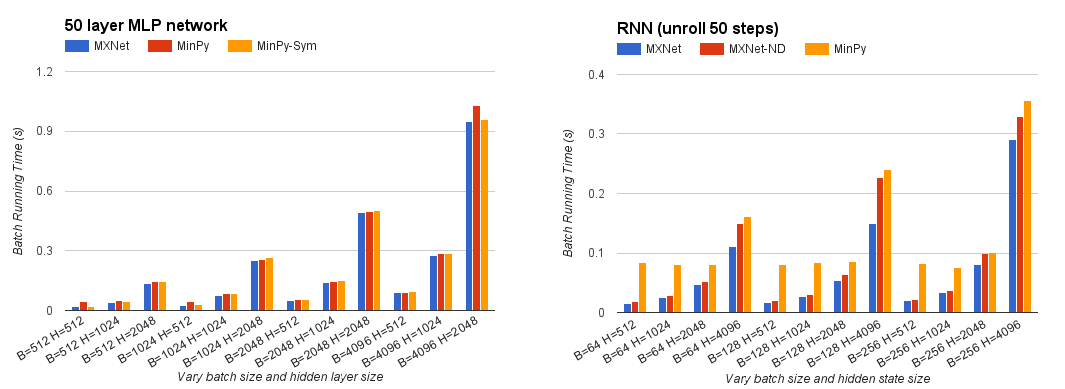Contributors
Last Commit
May. 15, 2019
Created
Nov. 5, 2015

# MinPy

Please try MXNet Gluon. Our project has merged with MXNet Gluon which follows our vision. This repo is being deprecated.

This repository aims at providing a high performing and flexible deep learning platform, by prototyping a pure NumPy interface above MXNet backend. In one word, you get the following automatically with your NumPy code:

`import minpy.numpy as np`
• Operators with GPU support will be ran on GPU.
• Graceful fallback for missing operations to NumPy on CPU.
• Automatic gradient generation with Autograd support.
• Seamless MXNet symbol integration.

## Pure NumPy, purely imperative

Why obsessed with NumPy interface? First of all, NumPy is an extension to the Python programming language, with support for large, multi-dimensional arrays, matrices, and a large library of high-level mathematical functions to operate on these abstractions. If you just begin to learn deep learning, you should absolutely start from NumPy to gain a firm grasp of its concepts (see, for example, the Stanford's CS231n course). For quick prototyping of advanced deep learning algorithms, you may often start composing with NumPy as well.

Second, as an extension of Python, your implementation follows the intuitive imperative style. This is the only style, and there is no new syntax constructs to learn. To have a taste of this, let's look at some examples below.

### Printing and DebuggingIn symbolic programming, the control dependency before the print statement is required, otherwise the print operator will not appear on the critical dependency path and thus not being executed. In contrast, MinPy is simply NumPy, as straightforward as Python's hello world.

### Data-dependent branchesIn symbolic programming, the `lambda` is required in each branch to lazily expand the dataflow graph during runtime, which can be quite confusing. Again, MinPy is NumPy, and you freely use the if statement anyway you like.

Tensorflow is just one typical example, many other packages (e.g. Theano, or even MXNet) have similar problems. The underlying reason is the trade-off between symbolic programming and imperative programming. Codes in symbolic programs (Tensorflow and Theano) generates dataflow graph instead of performing concrete computation. This enables extensive optimizations, but requires reinventing almost all language constructs (like if and loop). Imperative programs (NumPy) generates dataflow graph along with the computation, enabling you freely query or use the value just computed.

In MinPy, we use NumPy syntax to ease your programming, while simultaneously achieving good performance.

## Dynamic automatic gradient computation

Automatic gradient computation has become essential in modern deep learning systems. In MinPy, we adopt Autograd's approach to compute gradients. Since the dataflow graph is generated along with the computation, all kinds of native control flow are supported during gradient computation. For example:

```import minpy
from minpy.core import grad

def foo(x):
if x >= 0:
return x
else:
return 2 * x

foo_grad = grad(foo)
print foo_grad(3)  # should print 1.0
print foo_grad(-1) # should print 2.0```

Here, feel free to use native `if` statement. A complete tutorial about auto-gradient computation could be found here.

## Elegant fallback for missing operators

You never like `NotImplementedError`, so do we. NumPy is a very large library. In MinPy, we automatically fallback to NumPy if some operators have not been implemented in MXNet yet. For example, the following code runs smoothly and you don't need to worry about copying arrays back and forth from GPU to CPU; MinPy handles the fallback and its side effect transparently.

```import minpy.numpy as np
x = np.zeros((2, 3))     # Use MXNet GPU implementation
y = np.ones((2, 3))      # Use MXNet GPU implementation
z = np.logaddexp(x, y)   # Use NumPy CPU implementation```

## Seamless MXNet symbol support

Although we pick the imperative side, we understand that symbolic programming is necessary for operators like convolution. Therefore, MinPy allows you to "wrap" a symbol into a function that could be called together with other imperative calls. From a programmer's eye, these functions is just as other NumPy calls, thus we preserve the imperative style throughout:

```import mxnet as mx
import minpy.numpy as np
from minpy.core import Function
# Create Function from symbol.
net = mx.sym.Variable('x')
net = mx.sym.Convolution(net, name='conv', kernel=(3, 3), num_filter=32, no_bias=True)
conv = Function(net, input_shapes={'x', (8, 3, 10, 10)}
# Call Function as normal function.
x = np.zeros((8, 3, 10, 10))
w = np.ones((32, 3, 3, 3,))
y = np.exp(conv(x=x, conv_weight=w))```

## Is MinPy fast?

The imperative interface does raise many challenges, especially it foregoes some of the deep optimization that only (currently) embodied in symbolic programming. However, MinPy manages to retain performance, especially when the actual computation is intense. Our next target is to get back the performance with advanced system techniques.## Get Started

### Installation Guide

MinPy depends on MXNet. In order to get up and running with MinPy you'll need to

1. Install MXNet for Python;

2. Install Minpy.

Please read installation guide for more details.

### MXNet version

Currently both MXNet and MinPy are going through rapid development. MinPy is not guaranteed to work with all MXNet versions. The minimum version required for MXNet is 0.9.2. To achieve the best performance, we recommend you download the MXNet from `engine` branch and build it from source. The following command would be useful:

``````git clone --recursive -b engine https://github.com/dmlc/mxnet.git
``````

Then use the instructions to build MXNet with python interface.

### NumPy version

Minpy prototypes a pure Numpy interface. To make the interface consistent, please make sure Numpy version >= 1.10.0 before install Minpy.

MXNet and Numpy could meet version conflicts if you are working with them on other projects. Our installation guide provides how to use virtualenv and virtualenvwrapper to resolve the issue.

### Easy installation

``````pip install minpy
``````

We are still actively polishing the package. You can look at this tutorial to understand its concept. Documents are hosted here.

Latest Releases
v0.3.4
May. 13 2017
v0.3.3
Mar. 23 2017
v0.3.2
Feb. 1 2017
v0.3.1
Jan. 21 2017
v0.3.0
Jan. 16 2017